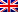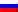# Planning problem

Contenus

## Planning and scheduling problem

Automated planning and scheduling is about carrying out strategies or sequences of actions. Modern planning, even within AI, has increasingly reflected the integration of theory and high-performance algorithmic techniques from operations research since at least the 1950s.

## Introduction to planning issues

Planning is the organization of the achievement of objectives in a specific area, with different means, and over a period / hierarchy. The result of this problem is a “plan” answering in detail the questions of the type QQOQCC: who, what, where, when, how and how much.

The planning problem makes it possible, depending on the conditions and the information, to answer the following questions:

• How to manage the logical sequence of tasks and distribute them over time?
• How to analyze the workloads requested from resources or means if they are limited?
• How to express a need for resources or means if they are not limited?
• How to take into account the constraints external to the project (customer order, supplier delivery, etc.)?
• How to analyze the consequences of a difference between actual and forecast events?
• How to identify late start or early end dates for activities?
• How to simulate optimistic, pessimistic, or most probable assumptions?
• How to analyze the impact of a hazard or risk if it turns into a problem?

The assignment problem is linked to many other problems in operations research (reduction of problems in the 21 Karp problems for example). Here we will see two sub-problems frequently encountered in assignment problems.

## Sub-problem: scheduling

The scheduling problem is a task scheduling problem in which it is a matter of deciding the order in which the tasks should be performed. This problem is Np-difficult taking into account the variety and the complexity of its constraints.

The different methods presented in this course make it possible to clearly and quickly show the data related to the realization of a plan, such as:

• means, or resources
• the costs.

The classic scheduling problem is as follows:

Let N tasks Ti requiring a duration ofi data and start date ti to be determined. The problem is subject to two types of constraints: time constraints - task duration and precedence; resource constraints - disjunctive: if tasks i and j are performed on machine k, they must be done in such and such an order; or cumulative: task i consumes aik of the resource k. The resource k has a capacity Ak. At all times, the sum of the consumptions on the machine k must be less than its capacity.

## Sub-problem: assignment

This problem is to best assign tasks to agents. Each agent can perform a single task for a given cost and each task must be performed by a single agent. The assignments (that is to say the agent-task pairs) all have a defined cost, the aim being to minimize the total cost of the assignments in order to carry out all the tasks. This problem is solved in polynomial time.

Given a set of agents S and a set of tasks T, it is possible to model the problem by a bipartite graph G = ((S, T), E), with a weight function vs on the edges. The assignment problem therefore consists in finding a perfect coupling F⊂E minimizing the sum ∑e∈F c (e) the weights of the edges of F.

## Sub-problem: transport

Like the two previous problems, the transport problem is a maximization. The transport problem is one of the subclasses of linear programming problems where the objective is to transport various quantities of a single homogeneous product which are initially stored at various origins, to different destinations such that the transport total is minimal.

Let ai the quantity of the commodity available at origin i; bj or the quantity of product required for destination j; vsij the cost of transporting one unit of a good from origin i to destination j; and xij is the quantity transported from origin i to destination j.

Here is the problem :

If the sum of the sources is equal to the sum of the demands, the problem is said to be balanced, in this case the constraints become equalities. Otherwise, we create a virtual demand point (dummy) corresponding to the excess supply and with a zero transport cost.

The transport problem is often represented as a bipartite graph with a flow problem (coupling). We will show here other variants to solve this problem.FRFRENRUES